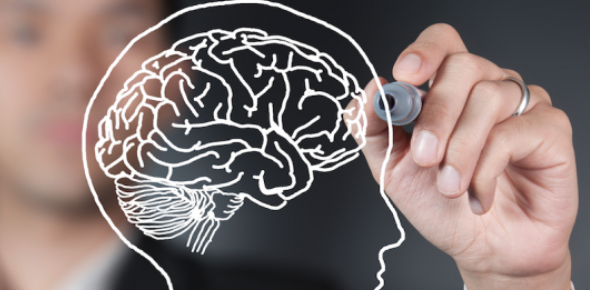# Psychology Quiz For Test

22 Questions | Total Attempts: 4924SettingsPsychology quiz for test.

• 1.
What is the scientific study of behavior and mental processes?
• A.

Biological perspective

• B.

Behavioral perspective

• C.

Psychology

• D.

Scientific method

• 2.
What is the application of the principles of evolution to explain psychological processes and phenomena?
• A.

Evolutionary perspective

• B.

Biological perspective

• C.

Psycho-dynamic perspective

• D.

Cognitive perspective

• 3.
Who was associated with the psychoanalysis theory and form of psychotherapy that emphasize the role of psychotherapy that emphasize the role of unconscious factors in personality and behavior.
• A.

B. F. Skinner

• B.

William James

• C.

Sigmund Freud

• D.

Wilhelm Wundt

• 4.
Who studied learning and effect of reinforcement?
• A.

B. F. Skinner

• B.

William James

• C.

Sigmund Freud

• D.

Wilhelm Wundt

• 5.
What are the physical aspects of behavior and mental processes that are studied by biological psychologists?
• A.

Evolutionary perspective

• B.

Cognitive perspective

• C.

Biological perspective

• D.

Behavioral perspective

• 6.
What focuses on important roles of mental processes in how people process and remember information?
• A.

Evolutionary perspective

• B.

Cognitive perspective

• C.

Biological perspective

• D.

Behavioral perspective

• 7.
What is a set of assumptions, altitudes, and procedures, that guide researchers in creating questions to investigate in generating evidence, and in drawing conclusions?
• A.

Case studies

• B.

Cross-culture perspective

• C.

Correlation studies

• D.

Scientific method

• 8.
What are the steps of the scientific method?
• 9.
What is a systematic observation and recording of behaviors as they occur in their natural setting
• A.

Correlation studies

• B.

Naturalistic observation

• C.

Surveys

• D.

Case studies

• 10.
What is an intensive, in depth investigation of an individual or a small group of individuals?
• A.

Case study

• B.

Survey

• C.

Sample

• D.

Random selection

• 11.
What is a segment of the larger group or population?
• A.

Survey

• B.

Representative sample

• C.

Random selection

• D.

Sample

• 12.
What is a segment that very closely parallels the larger population being studied on relevant characteristics?
• A.

Survey

• B.

Representative sample

• C.

Random selection

• D.

Sample

• 13.
What are objects that are selected randomly from a larger group such that every group such that every group member has an equal chance of being included in study?
• A.

Sample

• B.

Random selection

• C.

Case studies

• D.

Representative sample

• 14.
What is the research strategy that allows the precise calculation of how strongly related two factors are to each other?
• A.

Correlation coefficient

• B.

Positive correlation

• C.

Correlational study

• D.

Negative correlation

• 15.
What is the numerical indicator of the strength of the relationship between factors?
• A.

Correlation coefficient

• B.

Positive correlation

• C.

Correlational study

• D.

Negative correlation

• 16.
What is the two factor vary in the same direction?
• A.

Correlation coefficient

• B.

Positive correlation

• C.

Correlational study

• D.

Negative correlation

• 17.
What is the two variables more in opposite directions?
• A.

Correlation coefficient

• B.

Positive correlation

• C.

Correlational study

• D.

Negative correlation

• 18.
What is the research method to demonstrate a cause and effect relationship between changes in one variable and the effect that is produced on another variable?
• A.

Random assignment method

• B.

Scientific method

• C.

Causality method

• D.

Experimental method

• 19.
What are purposely manipulated factors thought to produce change in an experiment.
• A.

Independent variables

• B.

Dependent variables

• C.

Expectancy effects

• D.

Placebo

• 20.
What are factors that are observed and measured for change in an experiment?
• A.

Independent variables

• B.

Dependent variables

• C.

Expectancy effects

• D.

Placebo

• 21.
What is the process of assigning participants to experimental conditions so that all participants have an equal chance of being assigned to any of the conditions or groups in the study?
• A.

Expectancy effects

• B.

Random assignment

• C.

Demand characteristics

• D.

Double-blind study

• 22.
What are the 5 key provisions of ethics?
Related TopicsBack to top